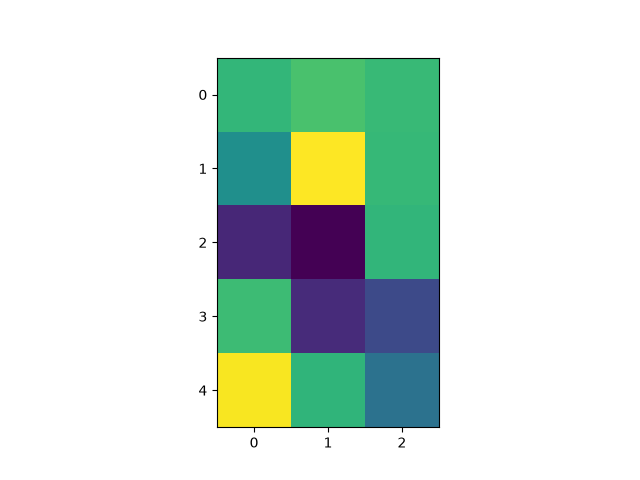# Modifying the coordinate formatter#

Modify the coordinate formatter to report the image "z" value of the nearest pixel given x and y. This functionality is built in by default; this example just showcases how to customize the format_coord function.

import matplotlib.pyplot as plt
import numpy as np

# Fixing random state for reproducibility
np.random.seed(19680801)

X = 10*np.random.rand(5, 3)

fig, ax = plt.subplots()
ax.imshow(X)

def format_coord(x, y):
col = round(x)
row = round(y)
nrows, ncols = X.shape
if 0 <= col < ncols and 0 <= row < nrows:
z = X[row, col]
return f'x={x:1.4f}, y={y:1.4f}, z={z:1.4f}'
else:
return f'x={x:1.4f}, y={y:1.4f}'

ax.format_coord = format_coord
plt.show()References

The use of the following functions, methods, classes and modules is shown in this example:

Gallery generated by Sphinx-Gallery